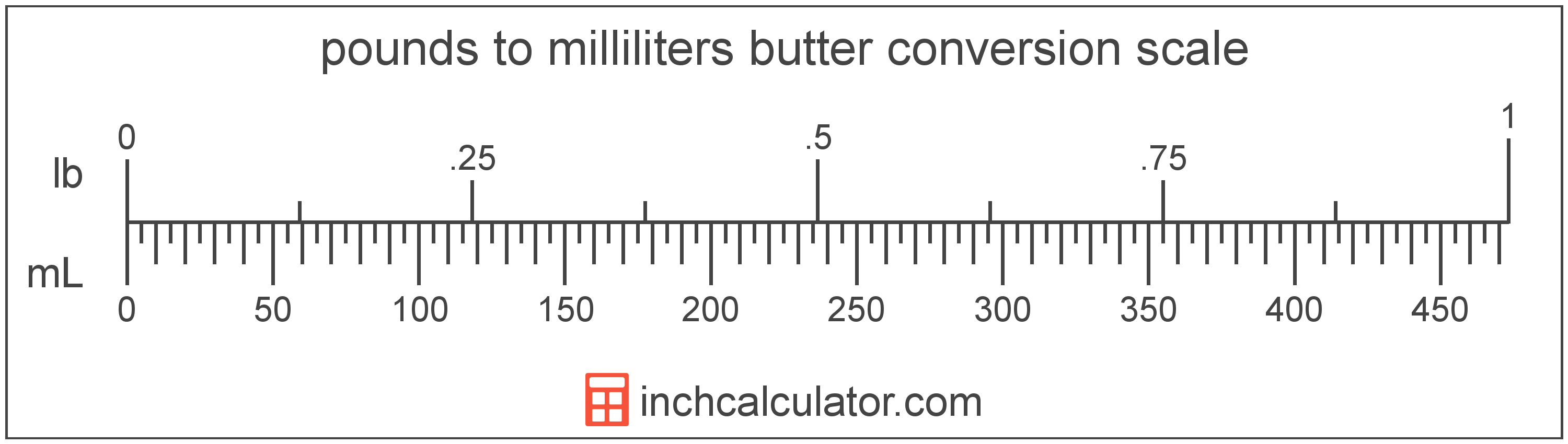# Convert Milliliters of Butter to Pounds

Enter the butter in milliliters below to get the value converted to pounds.

Results in Pounds:1 mL = 0.002113 lb

Do you want to convert pounds to milliliters?

## How to Convert Milliliters to Pounds

To convert a measurement in milliliters to a measurement in pounds, multiply the butter by the following conversion ratio: 0.002113 pounds/milliliter.

Since one milliliter of butter is equal to 0.002113 pounds, you can use this simple formula to convert:

pounds = milliliters × 0.002113

The butter in pounds is equal to the butter in milliliters multiplied by 0.002113.

For example, here's how to convert 500 milliliters to pounds using the formula above.
pounds = (500 mL × 0.002113) = 1.056688 lb## What Is a Milliliter?

Butter is sometimes measured by volume in milliliters. One stick of butter contains about 118.3 milliliters.

The milliliter is an SI unit of volume in the metric system. A milliliter is sometimes also referred to as a millilitre. Milliliters can be abbreviated as mL; for example, 1 milliliter can be written as 1 mL.

## What Is a Pound?

Butter in the US is most commonly sold by the pound, which contains 4 sticks, or 16 ounces.

The pound is a US customary and imperial unit of weight. Pounds can be abbreviated as lb; for example, 1 pound can be written as 1 lb.

## Milliliter to Pound Conversion Table

Table showing various milliliter measurements converted to pounds.
Milliliters Pounds
1 mL 0.002113 lb
2 mL 0.004227 lb
3 mL 0.00634 lb
4 mL 0.008454 lb
5 mL 0.010567 lb
6 mL 0.01268 lb
7 mL 0.014794 lb
8 mL 0.016907 lb
9 mL 0.01902 lb
10 mL 0.021134 lb
20 mL 0.042268 lb
30 mL 0.063401 lb
40 mL 0.084535 lb
50 mL 0.105669 lb
60 mL 0.126803 lb
70 mL 0.147936 lb
80 mL 0.16907 lb
90 mL 0.190204 lb
100 mL 0.211338 lb
200 mL 0.422675 lb
300 mL 0.634013 lb
400 mL 0.845351 lb
500 mL 1.0567 lb
600 mL 1.268 lb
700 mL 1.4794 lb
800 mL 1.6907 lb
900 mL 1.902 lb
1,000 mL 2.1134 lb